Courses

Test: Introduction & Properties of Fluid Level- 1

15 Questions MCQ Test Engineering Mechanics | Test: Introduction & Properties of Fluid Level- 1

Description
Attempt Test: Introduction & Properties of Fluid Level- 1 | 15 questions in 45 minutes | Mock test for Civil Engineering (CE) preparation | Free important questions MCQ to study Engineering Mechanics for Civil Engineering (CE) Exam | Download free PDF with solutions
QUESTION: 1

A 150 mm diameter shaft rotates at 1500 r. p. m. within a 200 mm long journal bearing with 150.5 mm internal diameter. The uniform annular space between the shaft and the bearing is filled with oil of dynamic viscosity 0.8 poise. The shear stress on the shaft will be

Solution: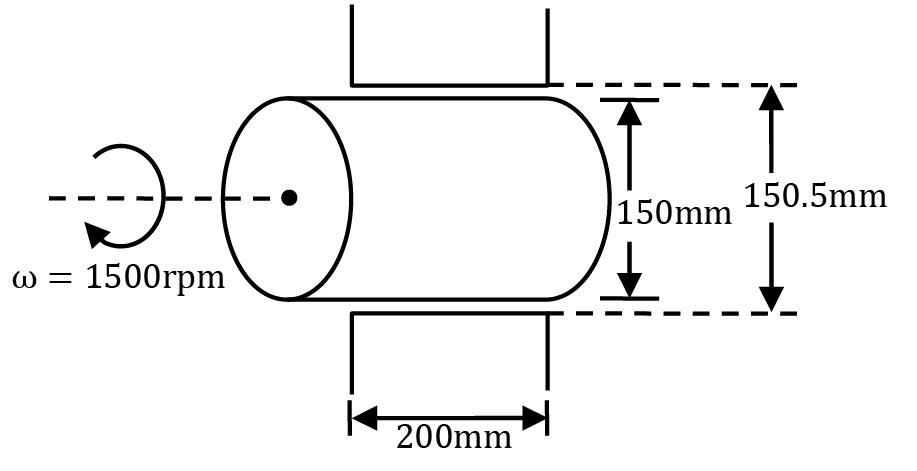As the shaft rotates, the oil in between the gap shall flow as shown. [Considering linear velocity distribution]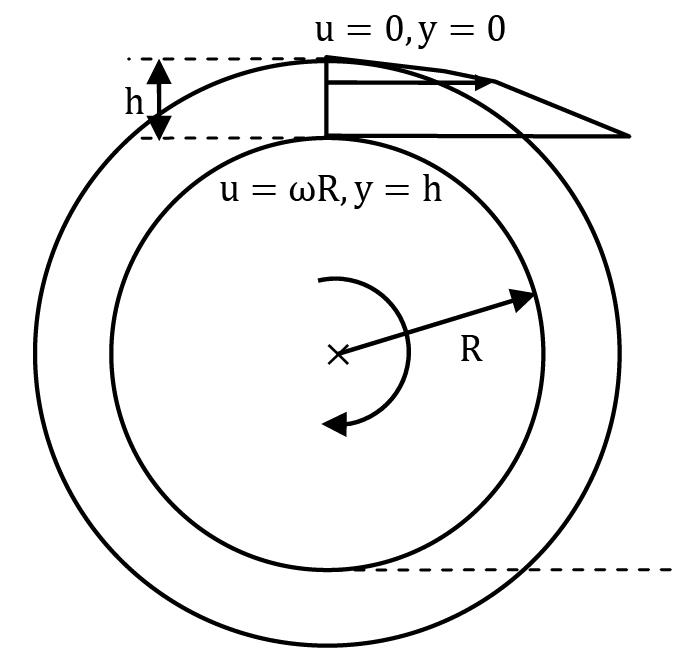Therefore τ = μ du / dy

τ = μ ( ωR / h )

τ = 0.8 / 10 × 1500 × 2π / 60 × 0.075 / (0.5 / 1000×2)

τ = 3769.91 Pa

or 3.769 kPa

Thus option (C) is the correct answer.

QUESTION: 2

What is the capillary rise in a narrow two dimensional slit of width ‘w’?

Solution: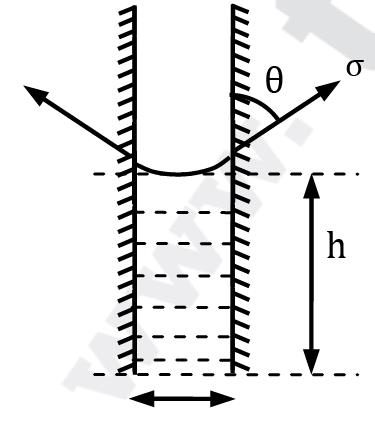Assume unit dimension perpendicular to the plane of paper.

W = FT

(wh × 1)ρg = (σ × 1) × cos θ The weight of the fluid that rises up in the slit is balanced by the vertical component of surface tension force.

⇒ h = 2σ cos θ wρg For a tube with diameter w We know h = 4σ cos θ ρgw Clearly the capillary rise in slit is 2 times less than tube.

QUESTION: 3

In an experiment to determine the rheological behavior of a material, the observed relation between shear stress, τ and rate of shear strain, du / dy is τ = τo + C ( du / dy )0.5 Which of the following represents variation of dynamic viscosity of fluid with rate of shear strain?

Solution:

By Ostwal-de Waal model

τ = ( du / dy )n + B

μapp = A ( du / dy )n-1

Given τ = τ0 + C ( du / dy )0.5

∴ μapp = C ( du / dy )-0.5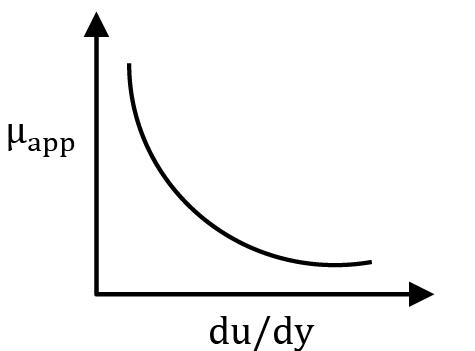Alternative Method: As per Ostwal-de Waal relationship, n < 1="" for="" the="" fluid,="" thus="" it="" is="" a="" pseudo="" plastic="" fluid.="" in="" such="" cases="" apparent="" viscosity="" decreases="" with="" increasing="" strain="" />

QUESTION: 4

A thin plane lamina of area A and weight W slides down a fixed plane inclined to the vertical at an angle α and maintains a uniform gap ε from the surface of the plane, the gap being filled with oil of viscosity μ. The terminal velocity of the plane is

Solution: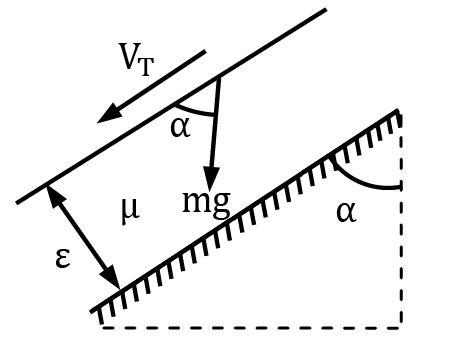As the plate starts sliding down because of its weight component, the oil between the plate and plane shall start flowing and viscous stress will act on the plate. With acceleration the velocity of plate and thus deformation rate of oil will increase. After the initial period of acceleration the viscous force will balance the weight component and plate will achieve equilibrium moving with constant velocity.

This final constant velocity is called terminal velocity. ∴ Gravitational force = Viscous Force

⇒ mg cos α = μ × VT / ε × A

⇒ given mg = W; we get VT = ε W cos α μA

QUESTION: 5

The figure shows a U-tube having a 5 mm × 5 mm square cross-section filled with mercury (specific gravity=13.6) up to a height of 20 cm in each limb (open to the atmosphere).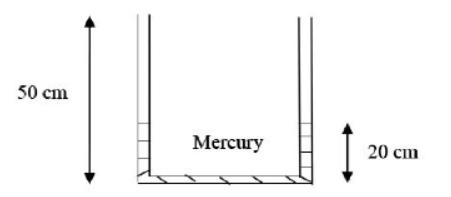If 5cm3 of water is added to the right limb, the new height (in cm, up to two decimal places) of mercury in LEFT limb will be __________

Solution: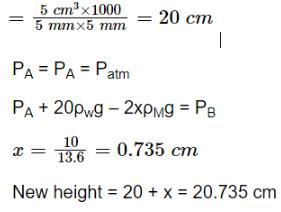QUESTION: 6

A spherical water drop of 1 mm in diameter splits up in air into 64 smaller drops of equal sizes. The surface tension coefficient of the water air interface is 0.073 N/m. The work required in splitting up the drop is

Solution: Imagine a small line of length dx on the surface of liquid with surface tension σ The force on the line = σdx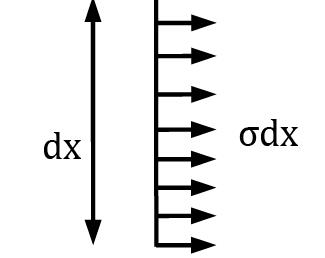If this line is moved by a distance dy perpendicular to its length.

The area generated = dx × dy

The work required to increase the

Area = Fsurface × dy = σdxdy = σdA

i. e The work required = σ × (Increase of area) So , !rea of 1mm dia drop

= 4π (0.5 × 10-3)2 = 3.14 × 10-6m

Volume of each droplet = 4 / 3 πr3 / 64 = πR3 / 48

r = [ 3 / 4π (πR3 / 48)1/3

r = 0.125 mm

Surface area of all 64 drops = 64 × 4 × π (0.125 × 10-3)2 = 1.25 × 10−5m2 Increase in surface area = 1.25 × 10−5 − 3.14 × 10-6 = 9.36 × 10-6

The work required

= σ × ΔA = 0.073 × 9.36 × 10-6

= 6.83 × 10-7J

= 0.68 × 10-6J

QUESTION: 7

The velocity distribution over a flat plate is gives by

u = 3 / 4y − y2

The shear stress at a location 0.3 m above the plate is k times the shear stress at a location 0.2 m above the plate. The value of k is

Solution: From Newton’s law of viscosity,

τ = μ du / dy

Given, u = 3 / 4y − y2

⇒ du / dy = 3 / 4− 2y

∴ τ0.3 = μ ( 3 / 4 − 2(0.3))

= 0.15 μ

τ0.2 = μ (3 / 4− 2(0.2))

= 0.35 μ

According to question,

τ0.3 = k τ0.2

⇒ 0.15 μ = k × 0.35 μ

⇒ k = 3 / 7

Therefore option (C) is correct.

QUESTION: 8

A beaker is filled with a liquid up to the mark of one litre and weighed. The weight of the liquid is found to be 6.5 N. The specific gravity of the liquid will be

Solution:

Specific gravity(S) of a liquid is defined as the ratio of the density of the liquid(pl) to that of water(pw).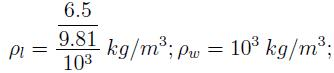Thus, S = 0:66.

QUESTION: 9

Develop a formula for capillary rise of a fluid having surface tension σ and contact angle θ between two concentric glass tubes of radii r0 and ri

Solution: Equating the weight of water risen to the vertical component of surface tension force,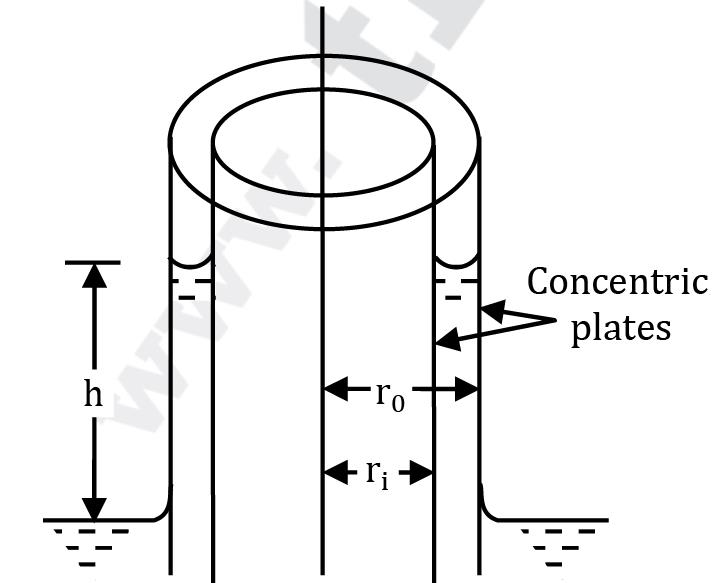wh π(r02 − ri2) = σ cos θ × 2π(r0 + ri)

∴ h = σ cos θ × 2π(r0 + ri) / wπ(r02 − r12)

= 2σ cos θ / w(r0 − ri)

QUESTION: 10

A beaker is filled with a liquid up to the mark of one litre and weighed. The weight of the liquid is found to be 6.5 N. The specific gravity of the liquid will be

Solution:

Specific gravity(S) of a liquid is defined as the ratio of the density of the liquid(pl) to that of water(pw).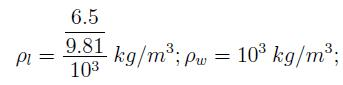Thus, S = 0.66.

QUESTION: 11

A circular disk of radius R is kept at a small height h above a fixed bed by means of a layer of oil of dynamic viscosity μ. If the disk is rotated at an angular velocity ω, obtain an expression for the viscous torque on the disk. Assume linear variation of velocity within the film.

Solution: Consider an element of disk of width dr at a radial distance r. For linear variation of velocity with depth h of oil film,

Shear stress τ = μ du / dy = μ u / h

Shear force = shear stress × area

= μ u / h × 2π r dr

Viscous torque on the element,

dT = μ u / h (2π r dr)r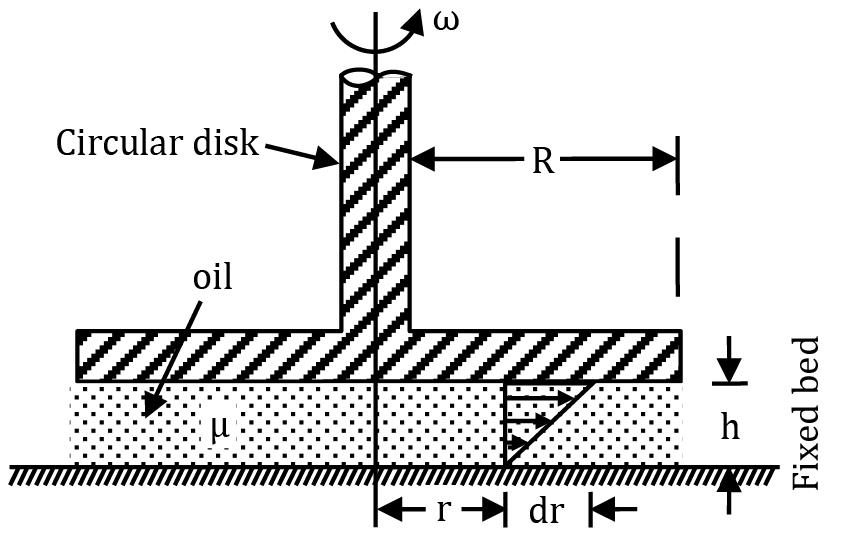Since the shaft rotates with angular velocity ω radians per second, the peripheral velocity u = ω r dT = μ ωr h (2π r dr)r = 2π μ ωr3dr h

Total viscous torque required to overcome the shearing force can be obtained by integrating from r = 0 to r = R.

Total torque T = 2π μ ω / h ∫R0 r3 dr

= 1 / 2 π μ ω / h R4

QUESTION: 12

A pressure vessel initially empty has an internal volume of 0.5 m3 . It is desired to test the vessel at 300 bar by pumping water into it. The estimated increase in volume of containers during the test is 6%. How much water (in kg) is required to be pumped? Consider the bulk modulus of elasticity for water to be 2 GPa.

Solution:

During the test, initial volume of container (when it is empty) is

V1 = 0.5 m3

The volume increases by 6%, thus final volume is

V2 = V1 + 6% of V1 = 0.5 × 1.06

= 0.53 m3

Let the water pumped into the container have a volume of V3 when it is outside (i.e. under atmospheric conditions). But when it is pumped into the container it’s final volume will be V2 and pressure equal to 300 bar.

∴ for water

K = dp / (−dV / V)

i. e. −dV / V = dp / K

⇒ −(V2 − V3) / V3 = dp / K

⇒ V3 − V2 / V3 = dp / K

⇒ V3 (1 − dp / K) = V2

⇒ V3 = V2 / 1 −dp / K

V3 = 0.53 / 1 −300×105 / 2×109

⇒ V3 = 0.538

Mass of water = ρ × V3 = 1000 × 0.538 = 538 kg

QUESTION: 13

In a 50 mm long journal-bearing arrangement, the clearance between the two concentric cylinders is 0.1 mm. The shaft is 20 mm in diameter and rotates at 3000 rpm. The dynamic viscosity of the lubricant used is 0.01 Pa s and the velocity variation in the lubricant is linear. Considering the lubricant to be Newtonian, calculate the frictional torque the journal has to overcome, and the corresponding power loss.

Solution:

For a linear velocity profile

du / dy =u / t

where u is the tangential velocity of the shaft and t is the thickness of oil film.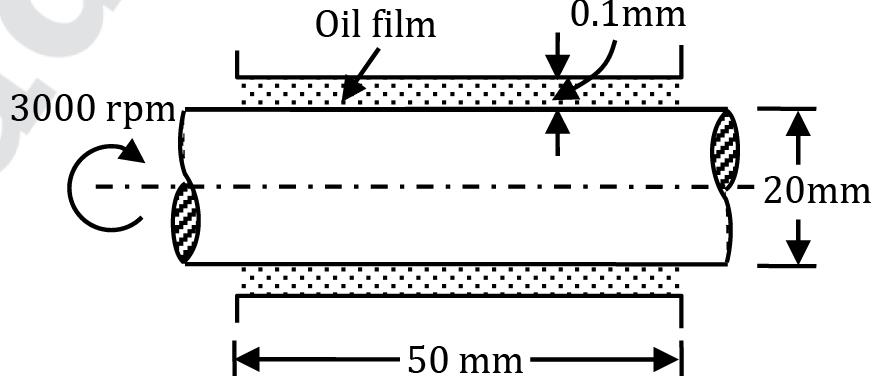Viscous shear stress τ = μ du / dy = μ u / t

Viscous resistance or force on the bearing

= shear stress × area

= μ u / t × 2πrl

Viscous torque = viscous force × torque arm

= μ u / t (2π rl)r

Given: μ = 0.01 Pa s = 0.01 N s/m2

r = 10 mm = 0.01 m ; l = 50 mm = 0.05 m

u = 2π rN / 60 = 2π × 0.01 × 3000 / 60

= 3.14 m/s

t = 0.1 mm = 0.0001 m

∴ Frictional torque,

T = 0.1 ×3.14 / 0.0001 × (2π × 0.01 × 0.05) × 0.01

= 9.85 × 10-2 Nm

If the shaft rotates with angular velocity

ω, than

ω = 2πN / 60 = 2π × 3000 / 60

∴ Power utilized in overcoming the frictional resistance,

P = T ω = (9.85 × 10-2) × 314

= 30.93 Nm/s = 30.93 W

QUESTION: 14

A hydraulic lift used for lifting automobiles has 20 cm diameter ram which slides in a 20.016 cm diameter cylinder. The annular space between the cylinder and ram is filled with an oil of kinematic viscosity 3.5 strokes and relative density 0.85. If the travel of 3.2 m long ram has a uniform rate of 15 cm/s, estimate the frictional resistance experienced by the ram.

Solution: Kinematic viscosity

ν = 3.5 stokes = 3.5 cm2/s = 3.5 × 10−4 m2/s

Mass density ρ = 0.85 × 1000 = 850 kg/m3

∴ Dynamic viscosity μ = ρ ν

= 850 × (3.5 × 10-4)

= 0.2975 N s/m2

Thickness of oil film

=(20.016 − 20) / 2 × 10-2

= 0.00008 m

Shear stress τ = μ du / dy = μ V / t

= 0.2975 × 0.15 / 0.00008 m

= 557.81 N/m2

Frictional resistance

= shear stress × area

= 557.81 × (π × 0.20 × 3.2)

= 1121 N = 1.12 kN

QUESTION: 15

n an experiment, the following shear stress time rate of shear strain values are obtained for a fluid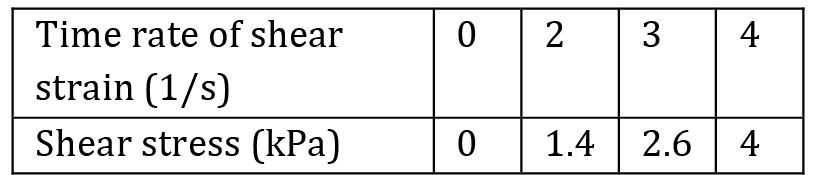Solution: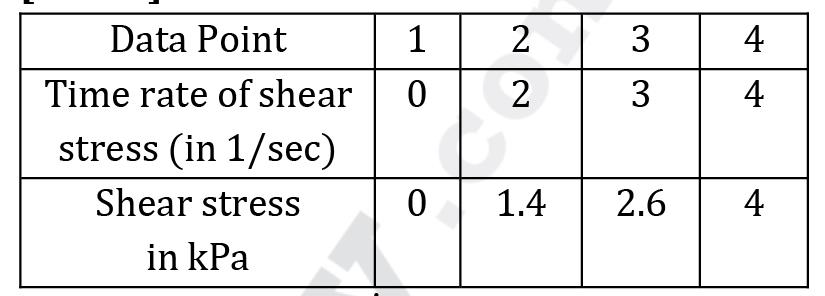The slope of τ vs ϕ̇ is equal to apparent viscosity of fluid.

Consider average slope (average apparent viscosity)

1. Data points ② and ①

μapp = 1.4 − 0 / 2 − 0 = 0.7

2. Data points ③ and ②

μapp = 2.6 − 1.4 / 3 − 2 = 1.2

3. Data points ④ and ③

μapp = 4 − 2.6 / 4 − 3 = 1.4

Thus as τ increases μapp also increases i.e. the fluid is shear thickening fluid (dilatant).Use Code STAYHOME200 and get INR 200 additional OFF Use Coupon Code

Track your progress, build streaks, highlight & save important lessons and more!

Similar Content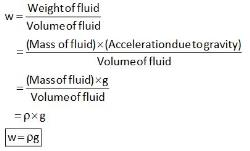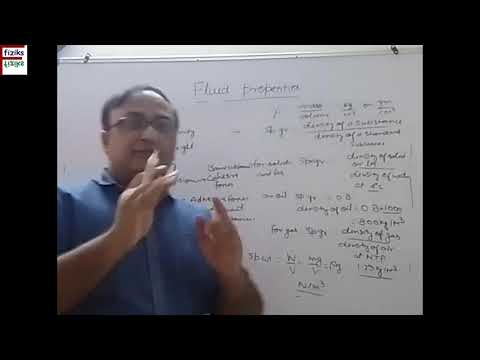Related tests